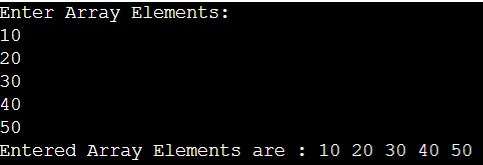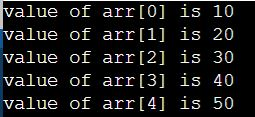# One Dimensional Array in C

## One Dimensional Array in C with Examples

In this article, I am going to discuss One Dimensional Array in C Language with Examples. Please read our previous articles, where we discussed the basics of Array in C Language.

##### One Dimensional Array in C:

One dimensional array is an array that has only one subscript specification that is needed to specify a particular element of an array. A one-dimensional array is a structured collection of components (often called array elements) that can be accessed individually by specifying the position of a component with a single index value.

Syntax: data-type arr_name[array_size];

##### Rules for Declaring One Dimensional Array
1. An array variable must be declared before being used in a program.
2. The declaration must have a data type(int, float, char, double, etc.), variable name, and subscript.
3. The subscript represents the size of the array. If the size is declared as 10, programmers can store 10 elements.
4. An array index always starts from 0. For example, if an array variable is declared as s, then it ranges from 0 to 9.
5. Each array element is stored in a separate memory location.
##### Initialization of One-Dimensional Array in C

An array can be initialized at the following states:

1. At compiling time (static initialization)
2. Dynamic Initialization
##### Compiling time initialization:

The compile-time initialization means the array of the elements is initialized at the time the program is written or array declaration.

Syntax: data_type array_name [array_size]=(list of elements of an array);
Example: int n={0, 1, 2, 3, 4};

###### Program:
```#include<stdio.h>
int main()
{
int n={0, 1, 2, 3, 4};
printf("%d", n);
printf("%d", n);
printf("%d", n);
printf("%d", n);
printf("%d", n);
}```

Output: 0 1 2 3 4

##### Run time initialization:

Run time initialization means the array can be initialized at runtime. That means array elements are initialized after the compilation of the program.

###### Program:
```#include<stdio.h>
#include<conio.h>
void main ()
{
int a, i;
printf ("Enter Array Elements:\n");
for (i = 0; i < 5; i++)
{
scanf ("%d", &a[i]);	//Run time array initialization
}
printf ("Entered Array Elements are : ");
for (i = 0; i < 5; i++)
{
printf ("%d ", a[i]);
}
getch ();
}```
###### Output:##### Array declaration, initialization, and accessing

Array declaration syntax: data_type arr_name [arr_size];
Array initialization syntax: data_type arr_name [arr_size]=(value1, value2, value3,….);
Array accessing syntax: arr_name[index];

###### Example: Integer array example:

int age ;
int age={0, 1, 2, 3, 4};

age; /*0 is accessed*/
age; /*1 is accessed*/
age; /*2 is accessed*/

###### Example: Character array example:

char str;
char str={‘H’,‘a’,‘i’};
(or)
char str = ‘H’;
char str = ‘a’;
char str = ‘i;

str; /*H is accessed*/
str; /*a is accessed*/
str; /*i is accessed*/

###### Example of One Dimensional Array in C
```#include<stdio.h>
int main()
{
int i;
int arr = {10,20,30,40,50};
// declaring and Initializing array in C
//To initialize all array elements to 0, use int arr={0};
/* Above array can be initialized as below also
arr = 10;
arr = 20;
arr = 30;
arr = 40;
arr = 50; */
for (i=0;i<5;i++)
{
// Accessing each variable
printf("value of arr[%d] is %d \n", i, arr[i]);
}
}```
###### Output:##### Example:

Create an integer array with size 5 and then takes the values from the keyboard and store them in the array and display the elements.

```#include<stdio.h>
int main()
{
int arr;
int i;
printf("\n Enter the array elemnts : ");
for(i = 0; i<5; i++)
{
scanf("%d", &arr[i]);
}
printf("\n The array elemts are : ");
for(i = 0; i<5; i++)
{
printf(" %d ", arr[i]);
}
return 0;
}```
###### Output:##### Example:

Create an integer array with size 5 and then calculate the larger element of that array using the function.

```#include<stdio.h>
int max(int arr[], int size)
{
int r, i;
r = arr;
for(i = 1; i<size; i++)
{
if (arr[i] > r)
r = arr[i];
}
return r;
}
int main()
{
int arr;
int m, i;
printf("Enter the array elements : ");
for(i = 0; i<5; i++)
scanf("%d", &arr[i]);
m = max(arr, 5);
printf("The largest element is : %d", m);
return 0;
}```
###### Output:In parameter creation, it is not possible to create an entire array as an argument. In formal argument location if we constructed array syntax then it creates pointer variable only. In formal argument location when we have int arr[] syntax, then it creates pointer variable only and the syntax will indicate that it holds single dimensional array address.

##### Copying 1d arrays in C:

We have two arrays list1 and list2
int list1 = {2, 4, 6, 8, 10, 12};
int list2;

and we want to copy the contents of list1 to list2. For general variables (e.g. int x=3, y=5) we use simple assignment statement (x=y or y=x). But for arrays the following statement is wrong.
list2 = list1;

We must copy between arrays element by element and the two arrays must have the same size.

###### Example of Copying One Dimensional Array in C
```#include <stdio.h>
#define MAX_SIZE 100
int main()
{
int source[MAX_SIZE], dest[MAX_SIZE];
int i, size;
/* Input size of the array */
printf("Enter the size of the array : ");
scanf("%d", &size);
/* Input array elements */
printf("Enter elements of source array : ");
for(i=0; i<size; i++)
{
scanf("%d", &source[i]);
}
/*
* Copy all elements from source array to dest array
*/
for(i=0; i<size; i++)
{
dest[i] = source[i];
}
/*
* Print all elements of source array
*/
printf("\nElements of source array are : ");
for(i=0; i<size; i++)
{
printf("%d\t", source[i]);
}
/*
* Print all elements of dest array
*/
printf("\nElements of dest array are : ");
for(i=0; i<size; i++)
{
printf("%d\t", dest[i]);
}
return 0;
}```
###### Output:##### Points to Remember About Array in C:
1. An array is a derived data type in C that is constructed from the fundamental data type of the C language.
2. An array is a collection of similar types of values in a single variable.
3. In implementation when we required the ‘n’ number of the variable of a similar data type then we need to go for the array.
4. When we are working with an array always memory will be created in the contiguous memory location, so randomly we can access the data.
5. In an array, all elements will share the same name with a unique identification value called index.
6. Always array index will start from 0 and ends with size – 1.
7. When we are working with an array compile-time memory management will occur i.e. static memory allocation.
8. Always size of the array must be an unsigned integer value which should be greater than zero.